# 22 A case study

To make the ideas in the previous chapter concrete, here we’ll work through the process of building a new geom that looks like a spring. This is a carefully crafted example: you’re unlikely to actually want to use springs to visualise your data (so no geom already exists), and they’re just complicated enough to illustrate the most important parts of the process.

We’ll develop the extension in five phases:

1. We’ll start as simple as possible by using the existing geom_path(), pairing it with a new stat.
2. The new stat only allows fixed diameter and tension, so we’ll next allow these to be set as parameters.
3. A stat is a great place to start but has some fundamental restrictions so we’ll convert our work so far in to a proper geom.
4. Geoms can only use dimensions relative to the data, and can’t use absolute sizes like 2cm, so next we’ll show you how to draw the spring with grid.
5. We’ll finish up by providing a custom scale and legend to pair with the geom.

Once you’ve worked your way through this chapter, we highly recommend browsing the ggplot2 source code to look at how other stats and geoms are implemented. They will often be more complicated than what you need, but they’ll give you a sense of the things you can do.

## 22.1 What is a spring?

Developing an extension usually starts with idea of what to draw. In this case, we want to draw a spring between two points. To begin, we’ll need to consider how to draw a spring. There are probably many ways, but one simple approach is to draw a circle while moving the “pen” in one direction:

circle <- tibble(
x = sin(seq(0, 2 * pi, length.out = 100)),
y = cos(seq(0, 2 * pi, length.out = 100)),
index = 1:100,
type = "circle"
)
spring <- circle
spring$x <- spring$x + seq(0, 1.5, length.out = 100)
spring$type <- "spring" ggplot(rbind(circle, spring)) + geom_path( aes(x = x, y = y, group = type, alpha = index), show.legend = FALSE ) + facet_wrap(~ type, scales = "free_x")It is clear that simply continuing to trace the circle while moving along x will make the spring longer, and that the speed of the x-movement will control how tightly spiralled the spring is. This gives us two parameters for our spring: • The diameter of the circle. • The tension, how fast we move along x. Although we can be pretty sure this is not a physically correct parameterisation of a spring, it is good enough for us. At this point, it’s worthwhile to spend a little time thinking about how we might turn this into a geom. How will we specify the diameter? How do we keep the circles circular even as we change the aspect ratio of the plot? Can we map diameter and tension to variables in the data? Are they scaled aesthetic? Or they both simple values that must be the same for all springs in a layer? ## 22.2 Part 1: A stat When developing a new layer, you have a choice between developing a Stat or a Geom. Surprisingly, the decision not guided by whether you want to end up with geom_spring() or stat_spring() because plenty of Stat extensions are used via a geom_*() constructor. Instead, you need to consider what you’re doing: if you’re just drawing transformed data with pre-existing geom, then you can use a Stat. Stats are much easier to extend than Geoms as they are simply data-transformation pipelines. Here we’re drawing a path but circling around instead of going in straight line. This is good fit of Stat, because we can transform data and then use the existing GeomPath. ### 22.2.1 Building functionality When developing a new Stat it’s a good idea to first write the data transformation function. Here we need a function that takes a start and end point, a diameter, and a tension. We will define tension to mean “times of diameter moved per revolution minus one”, thus 0 will mean that it doesn’t move at all, and will be forbidden as it would not allow our spring to extend between two points. We’ll also use a parameter n to give the number of points used per revolution, defining the visual fidelity of the spring. create_spring <- function(x, y, xend, yend, diameter = 1, tension = 0.75, n = 50) { if (tension <= 0) { rlang::abort("tension must be larger than zero.") } if (diameter == 0) { rlang::abort("diameter can not be zero.") } if (n == 0) { rlang::abort("n must be greater than zero.") } # Calculate direct length of segment length <- sqrt((x - xend)^2 + (y - yend)^2) # Figure out how many revolutions and points we need n_revolutions <- length / (diameter * tension) n_points <- n * n_revolutions # Calculate sequence of radians and x and y offset radians <- seq(0, n_revolutions * 2 * pi, length.out = n_points) x <- seq(x, xend, length.out = n_points) y <- seq(y, yend, length.out = n_points) # Create the new data data.frame( x = cos(radians) * diameter/2 + x, y = sin(radians) * diameter/2 + y ) } One nice thing about writing this function is that we can immediately test it out to convince ourselves that the logic works: spring <- create_spring( x = 4, y = 2, xend = 10, yend = 6, diameter = 2, tension = 0.75, n = 50 ) ggplot(spring) + geom_path(aes(x = x, y = y))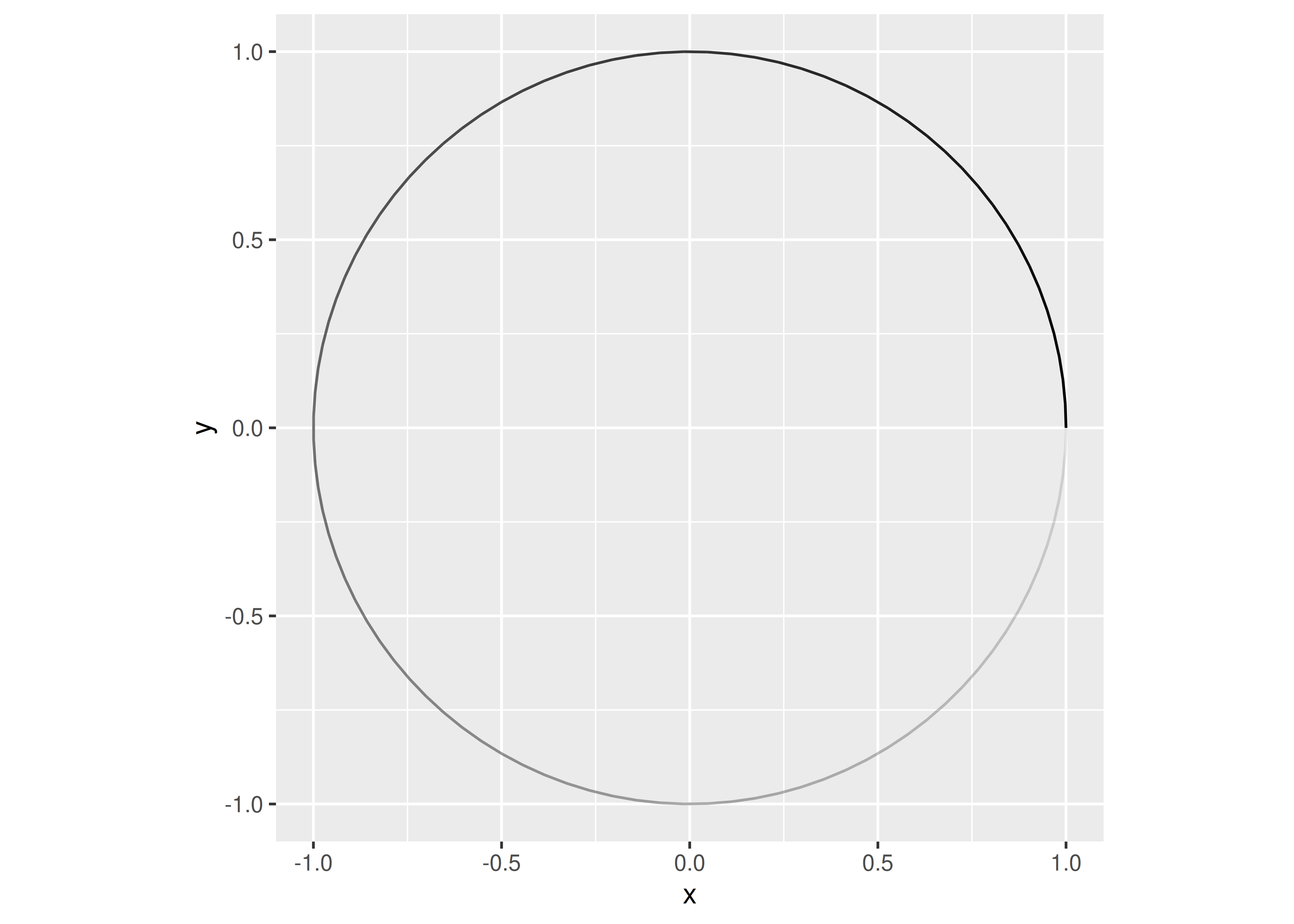(This would also be a great function to formally test with a unit testing package like testthat) Now we have the transformation function we can encapsulate it in a new Stat. We’ll define the Stat below and then work our way through each of the pieces. %||% <- function(x, y) { if (is.null(x)) y else x } StatSpring <- ggproto("StatSpring", Stat, setup_data = function(data, params) { if (anyDuplicated(data$group)) {
data$group <- paste(data$group, seq_len(nrow(data)), sep = "-")
}
data
},
compute_panel = function(data, scales,
diameter = 1,
tension = 0.75,
n = 50) {
cols_to_keep <- setdiff(names(data), c("x", "y", "xend", "yend"))
springs <- lapply(seq_len(nrow(data)), function(i) {
spring_path <- create_spring(
data$x[i], data$y[i],
data$xend[i], data$yend[i],
diameter = diameter,
tension = tension,
n = n
)
cbind(spring_path, unclass(data[i, cols_to_keep]))
})
do.call(rbind, springs)
},
required_aes = c("x", "y", "xend", "yend")
)

StatSpring <- ggproto("StatSpring", Stat,
...
)

This creates a new Stat52 subclass, named StatSpring. ggproto classes always use CamelCase for naming, and the new class is always saved into a variable with the same name.

### 22.2.2 Methods

Inside the class definition we implement methods by assigning functions to a name. You can see a complete list of methods by printing the class. The only methods that you shouldn’t touch are aesthetics and parameters; these are for internal use only. (To understand exactly what is passed to these methods we highly recommend adding a browser() statement)

print(Stat)
#> <ggproto object: Class Stat, gg>
#>     aesthetics: function
#>     compute_group: function
#>     compute_layer: function
#>     compute_panel: function
#>     default_aes: uneval
#>     extra_params: na.rm
#>     finish_layer: function
#>     non_missing_aes:
#>     optional_aes:
#>     parameters: function
#>     required_aes:
#>     retransform: TRUE
#>     setup_data: function
#>     setup_params: function

As discussed in the last chapter, the most important are the three compute_* methods. One of these must always be defined (and usually it’s the group or panel version). Here we use the compute_panel() method because it receives all the data for a single panel. As a rule of thumb, if the stat operates on multiple rows, start by implementing a compute_group() method, and if the stat operates on single rows, implement a compute_panel() method. Inside our compute_panel() method we do a bit more than simply call our create_spring() function.

StatSpring$compute_panel #> <ggproto method> #> <Wrapper function> #> function (...) #> f(...) #> #> <Inner function (f)> #> function(data, scales, #> diameter = 1, #> tension = 0.75, #> n = 50) { #> cols_to_keep <- setdiff(names(data), c("x", "y", "xend", "yend")) #> springs <- lapply(seq_len(nrow(data)), function(i) { #> spring_path <- create_spring( #> data$x[i], data$y[i], #> data$xend[i], data$yend[i], #> diameter = diameter, #> tension = tension, #> n = n #> ) #> cbind(spring_path, unclass(data[i, cols_to_keep])) #> }) #> do.call(rbind, springs) #> } We loop over each row of the data and create the points required to draw the spring. Then we combine our new data with all the non-position columns of the row. This is very important, since otherwise the aesthetic mappings to e.g. color and size would be lost. In the end we combine the individual springs into a single data frame that gets returned. Two other common methods are setup_data and setup_params which allows the class to do early checks and modifications of the parameters and data. Here our setup_data() method ensures that each input row has a unique group aesthetic. This is important because we’re going to draw our springs with GeomPath(), so we need to make sure that each row has it’s own id so the springs don’t get tangled. The group aesthetic is sometimes used to carry metadata so we preserve the existing value, and pasting on a unique id if needed. StatSpring$setup_data
#> <ggproto method>
#>   <Wrapper function>
#>     function (...)
#> f(...)
#>
#>   <Inner function (f)>
#>     function(data, params) {
#>     if (anyDuplicated(data$group)) { #> data$group <- paste(data$group, seq_len(nrow(data)), sep = "-") #> } #> data #> } The last part of our new class is the required_aes field. This is a character vector that gives the names of aesthetics that the user must provide to the stat. required_aes, along with default_aes and non_missing_aes, also defines the aesthetics that this stat understands. Any aesthetics that don’t appear in these fields (or in the fields in the corresponding geom) will generate a warning and the mapping will be ignored. StatSpring$required_aes
#>  "x"    "y"    "xend" "yend"

### 22.2.3 Constructors

Users never really see the ggproto objects (unless they go looking for them), since they are abstracted away into the well-known constructor functions that make up the ggplot2 API. Having created our stat, we should also create a constructor. A constructor isn’t strictly needed as geom_path(stat = "spring") will already work, but without a constructor there’s no good place to document our new functionality. Stat objects are almost paired with a geom_*() constructor because most ggplot2 users are accustomed to adding geoms, not stats, when building up a plot.

The constructor is mostly boilerplate; just take care to match the argument order and naming used in the ggplot2’s constructors so you don’t surprise your users.

geom_spring <- function(mapping = NULL,
data = NULL,
stat = "spring",
position = "identity",
...,
diameter = 1,
tension = 0.75,
n = 50,
arrow = NULL,
lineend = "butt",
linejoin = "round",
na.rm = FALSE,
show.legend = NA,
inherit.aes = TRUE
) {
layer(
data = data,
mapping = mapping,
stat = stat,
geom = GeomPath,
position = position,
show.legend = show.legend,
inherit.aes = inherit.aes,
params = list(
diameter = diameter,
tension = tension,
n = n,
arrow = arrow,
lineend = lineend,
linejoin = linejoin,
na.rm = na.rm,
...
)
)
}

Now that everything is in place, we can test out our new layer:

some_data <- tibble(
x = runif(5, max = 10),
y = runif(5, max = 10),
xend = runif(5, max = 10),
yend = runif(5, max = 10),
class = sample(letters[1:2], 5, replace = TRUE)
)

ggplot(some_data) +
geom_spring(aes(x = x, y = y, xend = xend, yend = yend))Because we’ve written a new stat, we get a number of features, like scaling and faceting, for free:

ggplot(some_data) +
geom_spring(
aes(x, y, xend = xend, yend = yend, colour = class),
linewidth = 1
) +
facet_wrap(~ class)For completion you should also create a stat constructor. Here our stat_spring() is very similar to geom_spring() except that it provides a default geom instead of a default stat.

stat_spring <- function(mapping = NULL, data = NULL, geom = "path",
position = "identity", ..., diameter = 1, tension = 0.75,
n = 50, na.rm = FALSE, show.legend = NA,
inherit.aes = TRUE) {
layer(
data = data,
mapping = mapping,
stat = StatSpring,
geom = geom,
position = position,
show.legend = show.legend,
inherit.aes = inherit.aes,
params = list(
diameter = diameter,
tension = tension,
n = n,
na.rm = na.rm,
...
)
)
}

We can test it out by drawing our springs with dots:

ggplot(some_data) +
stat_spring(
aes(x, y, xend = xend, yend = yend, colour = class),
geom = "point",
n = 15
) +
facet_wrap(~ class)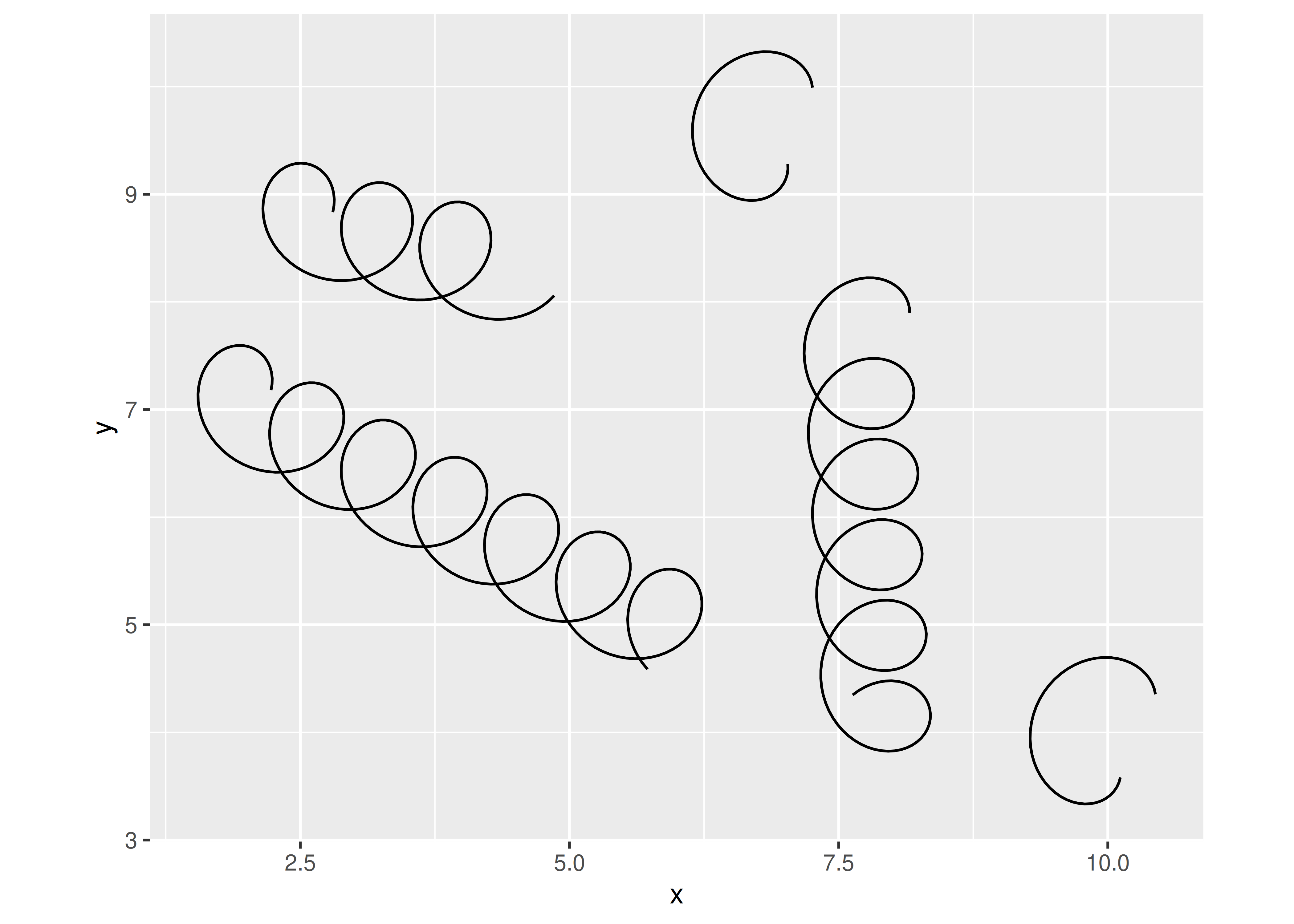### 22.2.4 Post-mortem

We have now successfully created our first extension. One shortcoming of our implementation is that diameter and tension are constants that can only be set for the full layer. These settings feel more like aesthetics and it would be nice if their values could be mapped to a variable in the data.

Another, potentially bigger, issue is that the spring path is relative to the coordinate system of the plot. This means that strong deviations from an aspect ratio of 1 will visibly distort the spring, as can be seen in the example below:

ggplot() +
geom_spring(aes(x = 0, y = 0, xend = 3, yend = 20))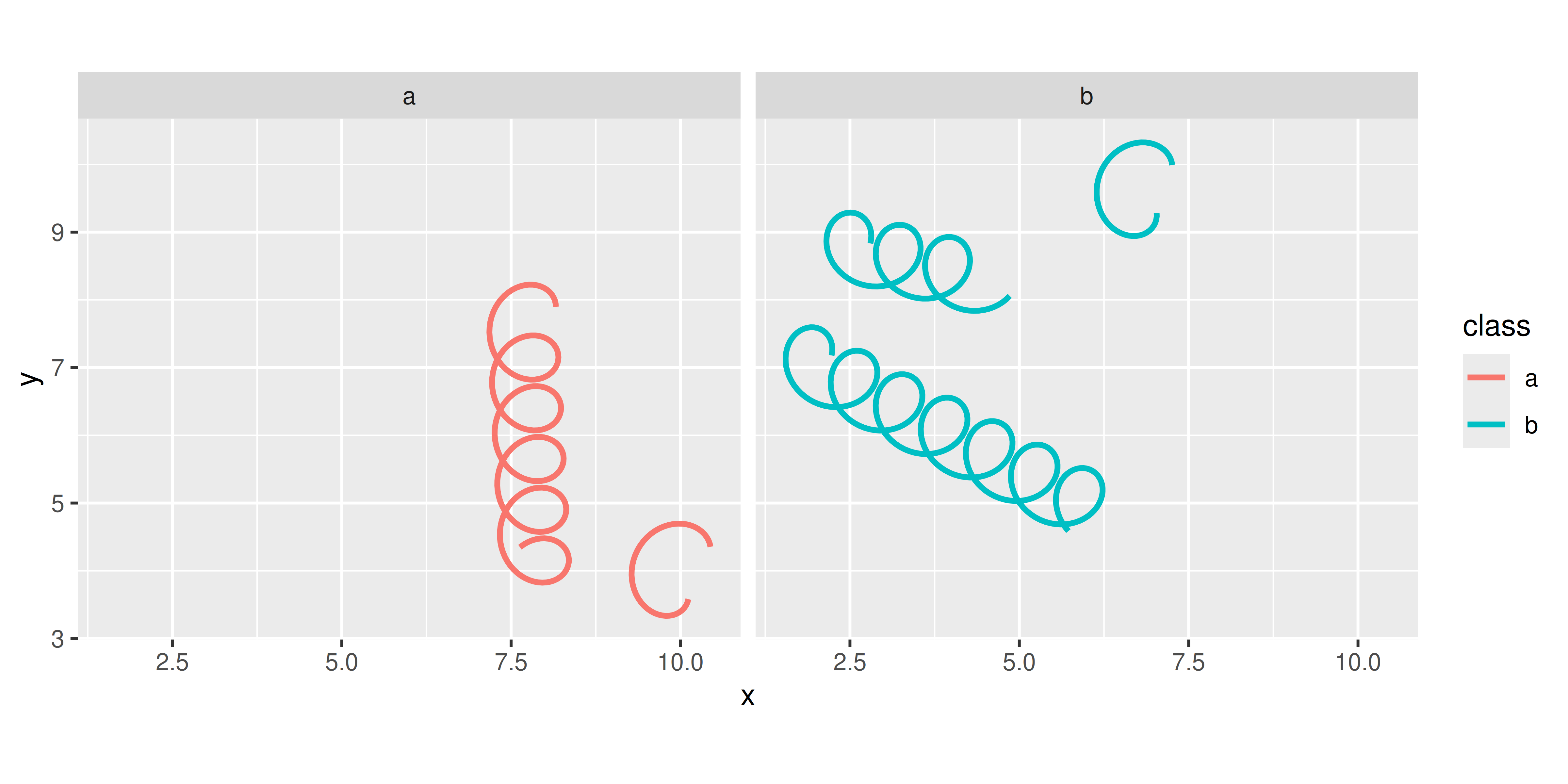The same underlying problem means that the diameter is expressed in coordinate space, meaning that it is difficult to define a meaningful default:

ggplot() +
geom_spring(aes(x = 0, y = 0, xend = 100, yend = 80))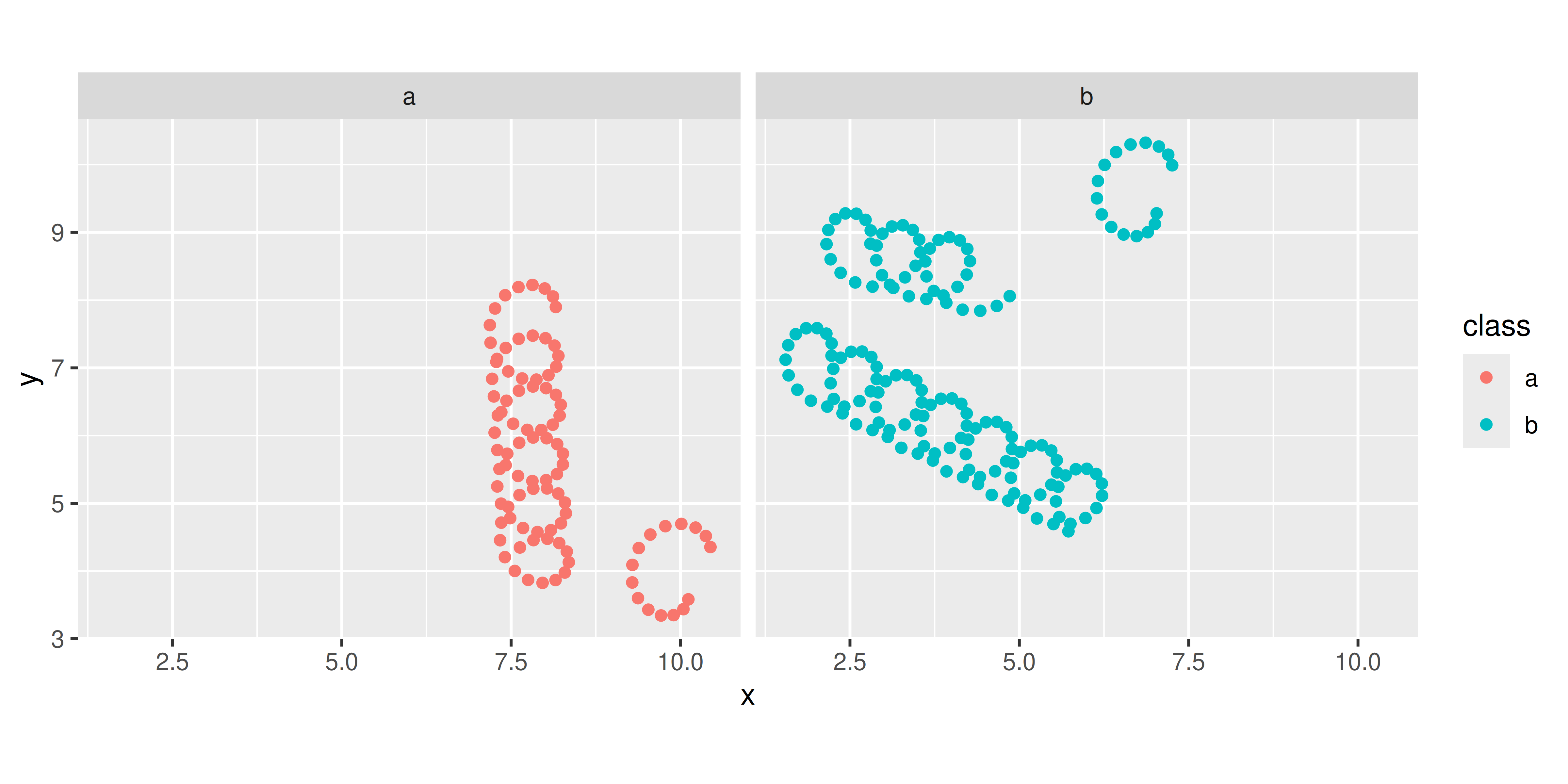## 22.3 Part 2: Adding aesthetics

We’ll tackle the first challenge by turning the diameter and tension arguments into aesthetics that can be set per-spring. There is surprisingly little to do here:

StatSpring <- ggproto("StatSpring", Stat,
setup_data = function(data, params) {
if (anyDuplicated(data$group)) { data$group <- paste(data$group, seq_len(nrow(data)), sep = "-") } data }, compute_panel = function(data, scales, n = 50) { cols_to_keep <- setdiff(names(data), c("x", "y", "xend", "yend")) springs <- lapply(seq_len(nrow(data)), function(i) { spring_path <- create_spring(data$x[i], data$y[i], data$xend[i],
data$yend[i], data$diameter[i],
data$tension[i], n) cbind(spring_path, unclass(data[i, cols_to_keep])) }) do.call(rbind, springs) }, required_aes = c("x", "y", "xend", "yend"), optional_aes = c("diameter", "tension") ) The main difference with our previous attempt is that the diameter and tension arguments to compute_panel() have gone away, and they’re now taken from the data (just like x, y, etc). This has a downside (that we’ll fix shortly): we can no longer set fixed aesthetics so we’ll also need to remove from the constructor: geom_spring <- function(mapping = NULL, data = NULL, stat = "spring", position = "identity", ..., n = 50, arrow = NULL, lineend = "butt", linejoin = "round", na.rm = FALSE, show.legend = NA, inherit.aes = TRUE) { layer( data = data, mapping = mapping, stat = stat, geom = GeomPath, position = position, show.legend = show.legend, inherit.aes = inherit.aes, params = list( n = n, arrow = arrow, lineend = lineend, linejoin = linejoin, na.rm = na.rm, ... ) ) } The stat_spring() constructor would require the same kind of change. All that is left is to test our new implementation out: some_data <- tibble( x = runif(5, max = 10), y = runif(5, max = 10), xend = runif(5, max = 10), yend = runif(5, max = 10), class = sample(letters[1:2], 5, replace = TRUE), tension = runif(5), diameter = runif(5, 0.5, 1.5) ) ggplot(some_data, aes(x, y, xend = xend, yend = yend)) + geom_spring(aes(tension = tension, diameter = diameter))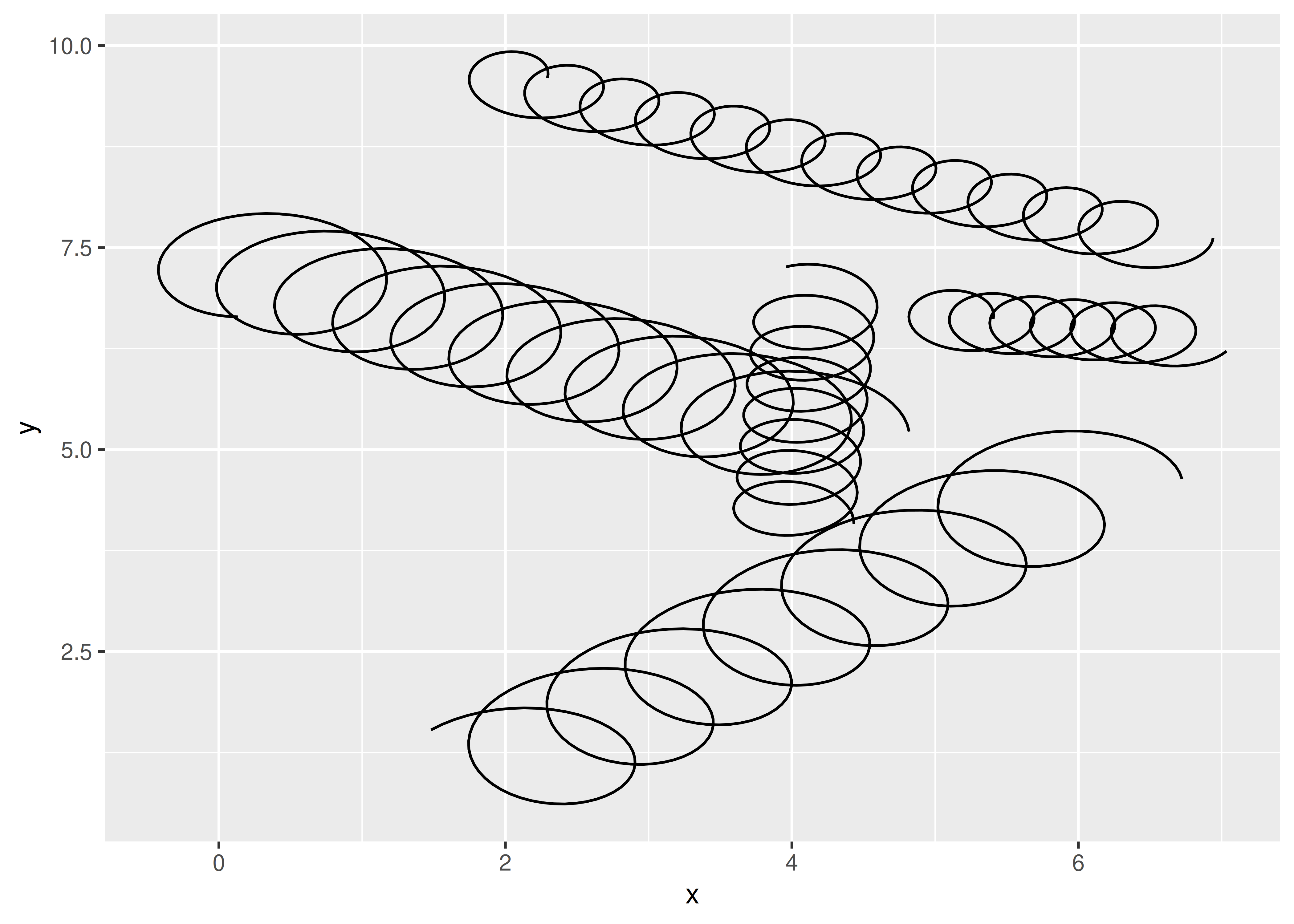It appears to work, we can no longer set diameter and tension as parameters: ggplot(some_data, aes(x, y, xend = xend, yend = yend)) + geom_spring(diameter = 0.5) #> Warning: Ignoring unknown parameters: diameter #> Warning: Computation failed in stat_spring(): #> argument is of length zero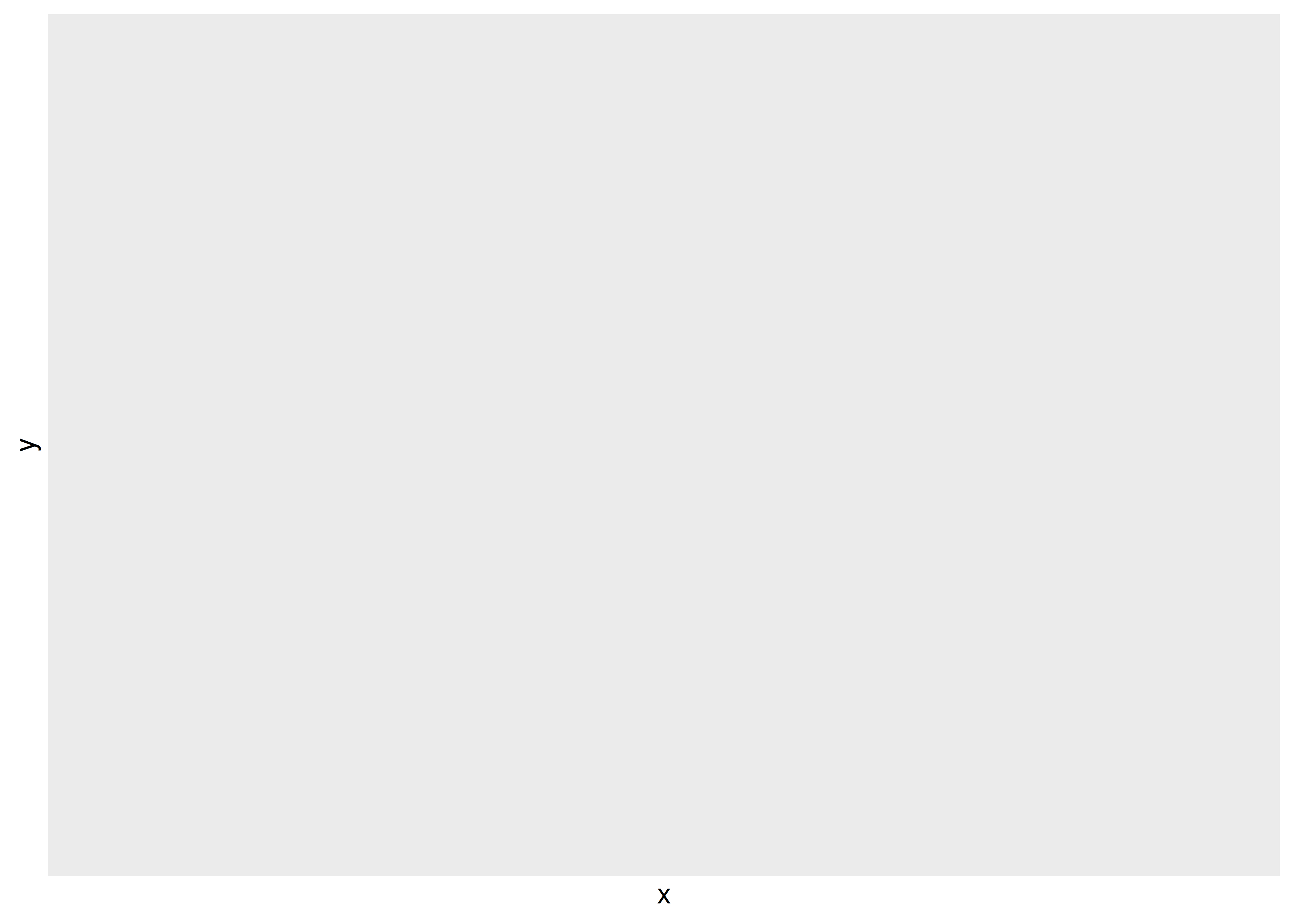### 22.3.1 Post-Mortem In this section we further developed our spring stat so that diameter and tension can be used as aesthetics, varying across springs. Unfortunately, there’s a major downside: these features no longer can be set globally. We’re still also missing a way to control the scaling of the two aesthetics. Fixing both these problems requires the same next step: move our implementation away from Stat and towards a proper Geom. ## 22.4 Part 3: A geom In many cases a Stat-centred approach is sufficient, for example, many of the graphic primitives provided by the ggforce package are Stats. But we need to go further with the spring geom because the tension and diameter aesthetics need specified in units that are unrelated to the coordinate system. Consequently, we’ll rewrite our geom to be a proper Geom extension. ### 22.4.1 Geom extensions As discussed in Section 21, there are many similarities between Stat and Geom extensions. The biggest difference is that Stat extensions return a modified version of the input data, whereas Geom extensions return graphical objects (technically, grid grobs; more on that later). Since our geom is a special type of a path, we can get pretty far by simply extending GeomPath and modifying the data before it is rendered: GeomSpring <- ggproto("GeomSpring", GeomPath, ..., setup_data = function(data, params) { cols_to_keep <- setdiff(names(data), c("x", "y", "xend", "yend")) springs <- lapply(seq_len(nrow(data)), function(i) { spring_path <- create_spring( data$x[i], data$y[i], data$xend[i], data$yend[i], diameter = data$diameter[i],
tension = data$tension[i], n = params$n
)
spring_path <- cbind(spring_path, unclass(data[i, cols_to_keep]))
spring_path$group <- i spring_path }) do.call(rbind, springs) }, ... ) Here we override the the setup_data() method, applying create_spring() to each row. This is simple, but isn’t really an improvement over our StatSpring approach because setup_data() is called before the default and set aesthetics are added to the data, so it will only work if everything is defined within aes(). To make things better, we’ll need to move our data manipulation into the draw_*() methods. Fortunately, we can re-use the GeomPath implementation so we don’t yet have to learn about exactly what type of output we need to produce: GeomSpring <- ggproto("GeomSpring", Geom, setup_data = function(data, params) { if (is.null(data$group)) {
data$group <- seq_len(nrow(data)) } if (anyDuplicated(data$group)) {
data$group <- paste(data$group, seq_len(nrow(data)), sep = "-")
}
data
},
draw_panel = function(data, panel_params, coord, n = 50, arrow = NULL,
lineend = "butt", linejoin = "round", linemitre = 10,
na.rm = FALSE) {
cols_to_keep <- setdiff(names(data), c("x", "y", "xend", "yend"))
springs <- lapply(seq_len(nrow(data)), function(i) {
spring_path <- create_spring(data$x[i], data$y[i], data$xend[i], data$yend[i], data$diameter[i], data$tension[i], n)
cbind(spring_path, unclass(data[i, cols_to_keep]))
})
springs <- do.call(rbind, springs)
GeomPath$draw_panel( data = springs, panel_params = panel_params, coord = coord, arrow = arrow, lineend = lineend, linejoin = linejoin, linemitre = linemitre, na.rm = na.rm ) }, required_aes = c("x", "y", "xend", "yend"), default_aes = aes( colour = "black", linewidth = 0.5, linetype = 1L, alpha = NA, diameter = 1, tension = 0.75 ) ) Developers used to object-oriented design may frown upon this design where we call the method of another kindred object directly (GeomPath$draw_panel()), but since Geom objects are stateless this is as safe as subclassing GeomPath and calling the parent method. You can see this approach all over the place in the ggplot2 source code.

If you compare this code to our StatSpring implementation in the last chapter you can see that the compute_panel() and draw_panel() methods are quite similar with the main difference being that we pass on the computed spring coordinates to GeomPath$draw_panel() in the latter method. Our setup_data() method has been greatly simplified because we now relies on the default_aes functionality in Geom to fill out non-mapped aesthetics. Creating the geom_spring() constructor is almost similar, except that we now use the identity stat instead of our spring stat and use the new GeomSpring instead of GeomPath. geom_spring <- function(mapping = NULL, data = NULL, stat = "identity", position = "identity", ..., n = 50, arrow = NULL, lineend = "butt", linejoin = "round", na.rm = FALSE, show.legend = NA, inherit.aes = TRUE) { layer( data = data, mapping = mapping, stat = stat, geom = GeomSpring, position = position, show.legend = show.legend, inherit.aes = inherit.aes, params = list( n = n, arrow = arrow, lineend = lineend, linejoin = linejoin, na.rm = na.rm, ... ) ) } Without much additional work we now have a proper geom with working default aesthetics and the possibility of setting aesthetics as parameters. ggplot(some_data, aes(x, y, xend = xend, yend = yend)) + geom_spring(diameter = 0.5)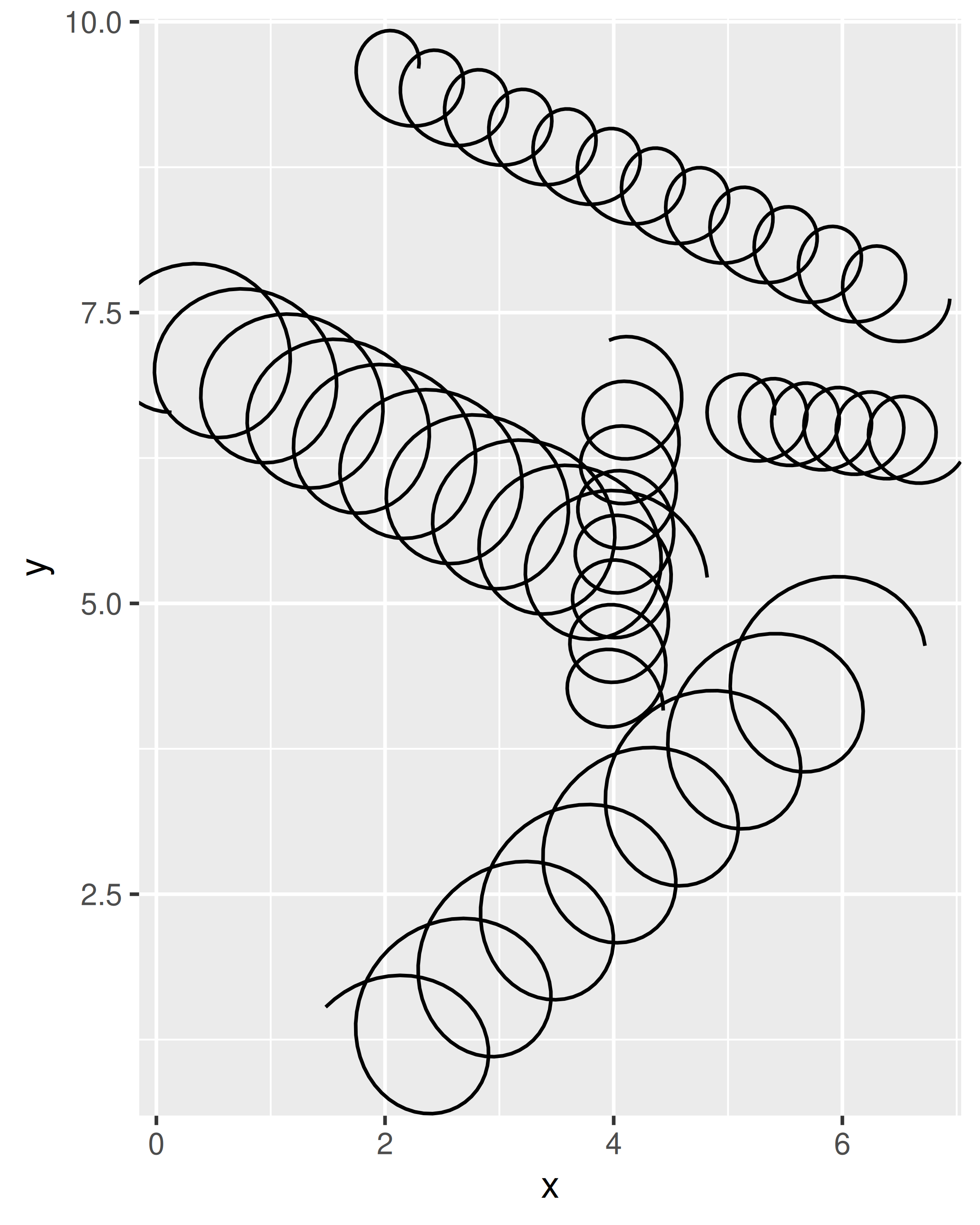This is basically as far as we can get without learning about grid grobs, the underlying object that actually does the drawing. Creating grid grobs is an advanced technique, needed by relatively few geoms. But creating a grid grob gives you the power to use absolute units (e.g. 1cm) and to adjust the display of the geom based on the size of the output device. ### 22.4.2 Post-Mortem In this section we finally created a full Geom extension that behaves as you’d expect. This is often, but not always, the natural conclusion to the development of new layer. The chief advantage of the Stat approach is that you can use the same stat with multiple geoms. The final choice is ultimately up to you and should be guided by how you envision the layer to be used. We haven’t talked about what goes on inside the draw_*() methods yet. Often it is enough to use a method from existing geom. For example, even the relatively component GeomBoxplot just uses the draw methods from GeomPoint(), GeomSegment and GeomCrossbar. But if you need to go deeper, you’ll need to learn a little about grid. ## 22.5 Part 4: A grid grob In the last section we exhausted our options for our spring geom safe for delving into the development of a new grid grob. grid is the underlying graphic system that ggplot2 builds upon and while much can be achieved by ignoring grid entirely, there are situations where it is impossible to achieve what you want without going down to the grid level. There are especially two situations that warrant the need for using grid directly when developing ggplot2 extensions: 1. You need to create graphical objects that are positioned correctly on the coordinate system, but where some part of their appearance has a fixed absolute size. In our case this would be the spring correctly going between two points in the plot, but the diameter being defined in cm instead of relative to the coordinate system. 2. You need graphical objects that are updated during resizing. This could e.g. be the position of labels such as in the ggrepel package or the geom_mark_*() geoms in ggforce. Before we begin developing the new version of our geom it will be good to have at least a cursory understanding of the key concepts in grid: ### 22.5.1 Grid in 5 minutes There are two drawing systems included in base R: base graphics and grid. Base graphics has an imperative “pen-on-paper” model: every function immediately draws something on the graphics device. Grid takes a more declarative approach where you build up a nested description of the graphic as an object, which is later rendered (much like ggplot2). This gives us an object that exists independently of the graphic device and can be passed around, analysed, and modified. Importantly, parts of the object can refer to other parts, so that you can say (e.g.) make this rectangle as wide as that string of text. The next few sections will give you the absolute minimum vocabulary to understand ggplot2’s use of grid: grobs, viewports, and units. To get a sufficient understanding of grid to create your own geoms, we highly recommend Paul Murrell53. #### 22.5.1.1 Grobs Grobs (graphic objects) are the atomic representations of graphical elements in grid, and include types like points, lines, rectangles, and text. All grid objects are vectorised, so that (e.g.) a point grob can represent multiple points. As well as simple geometric primitive, grid also comes the means to combine multiple grobs into a complex objects called gTree()s. You can create a new grob class with the grob() or gTree() constructors, and then give it special behaviour by override the makeContext() and makeContent() S3 generics: • makeContext() is called when the parent grob is rendered and allows you to control the viewport of the grob (see below). • makeContent() is called everytime the drawing region is resized and allows you to customise the look based on the size or other aspect. #### 22.5.1.2 Viewports grid viewports define rectangular plotting regions. Each viewport defines a coordinate system for position grobs, and optionally, a tabular grid that child viewports can occupy. A grob can have its own viewport or inherit the viewport of its parent. While we won’t need to consider viewports for our spring grob, they’re an important concept that powers much of the high-level layout of ggplot2 graphics. #### 22.5.1.3 Units grid units provide a flexible way of specifying positions (e.g. x and y) and dimensions (e.g. length and width) of grobs and viewports. There are three types of unit: • Absolute units (e.g. centimeters, inches, and points). • Relative units (e.g. npc which scales the viewport size between 0 and 1). • Units based on other grobs (e.g. grobwidth). units support arithmetic operations and are only resolved at draw time so it is possible to combine different types of units. For example unit(0.5, "npc") + unit(1, "cm") defines a point one centimeter to the right of the center of the current viewport. #### 22.5.1.4 Example Given this very cursory introduction, let’s now look at an example grob. First, let’s load the grid package: library(grid) The code below will create a grob that appears as a square if bigger than 5 cm and a circle if smaller: surpriseGrob <- function(x, y, size, default.units = "npc", name = NULL, gp = gpar(), vp = NULL) { # Check if input needs to be converted to units if (!is.unit(x)) { x <- unit(x, default.units) } if (!is.unit(y)) { y <- unit(y, default.units) } if (!is.unit(size)) { size <- unit(size, default.units) } # Construct our surprise grob subclass as a gTree gTree( x = x, y = y, size = size, name = name, gp = gp, vp = vp, cl = "surprise" ) } makeContent.surprise <- function(x) { x_pos <- x$x
y_pos <- x$y size <- convertWidth(x$size, unitTo = "cm", valueOnly = TRUE)

# Figure out if the given sizes are bigger or smaller than 5 cm
circles <- size < 5

# Create a circle grob for the small ones
if (any(circles)) {
circle_grob <- circleGrob(
x = x_pos[circles],
y = y_pos[circles],
r = unit(size[circles] / 2, "cm")
)
} else {
circle_grob <- nullGrob()
}

# Create a rect grob for the large ones
if (any(!circles)) {
square_grob <- rectGrob(
x = x_pos[!circles],
y = y_pos[!circles],
width = unit(size[!circles], "cm"),
height = unit(size[!circles], "cm")
)
} else {
square_grob <- nullGrob()
}

# Add the circle and rect grob as childrens of our input grob
setChildren(x, gList(circle_grob, square_grob))
}

# Create an instance of our surprise grob defining to object with different
# sizes
gr <- surpriseGrob(x = c(0.25, 0.75), y = c(0.5, 0.5), size = c(0.1, 0.4))

# Draw it
grid.newpage()
grid.draw(gr)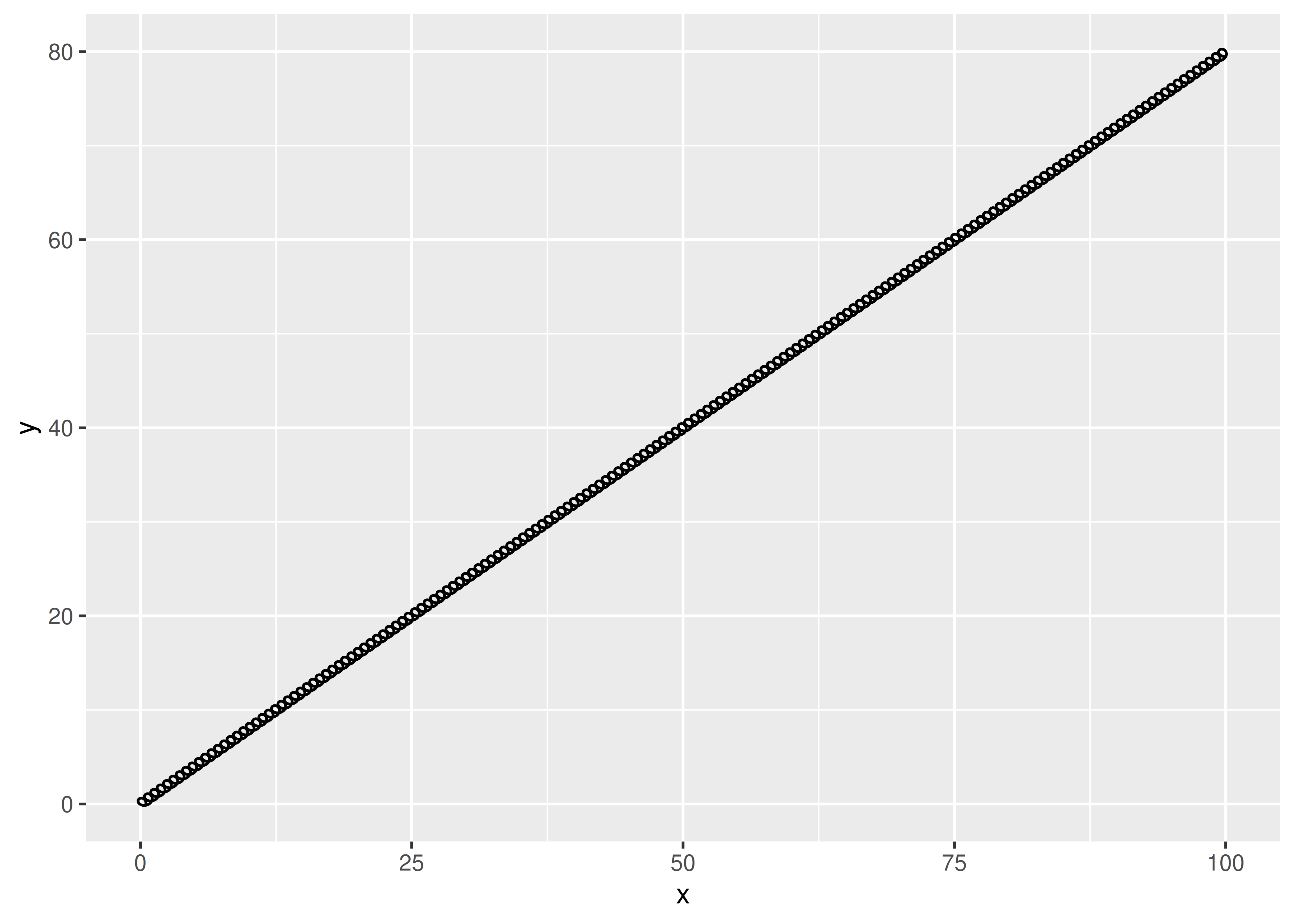If you run the code above interactively and resize the plotting window you can see that the two objects will change form based on the size of the plotting window. This is a useless example, of course, but hopefully you can see how this technique can be used to do real work.

### 22.5.2 The springGrob

With our new knowledge of the grid system we can now see how we might construct a spring grob that has an absolute diameter. If we wait with the expansion to the spring path until the makeContent() function, and calculate it based on coordinates in absolute units we can make sure that the diameter stays constant during resizing of the plot. With that in mind, we can create our constructor. We model the arguments after segmentsGrob() since we are basically creating modified segments:

springGrob <- function(x0 = unit(0, "npc"), y0 = unit(0, "npc"),
x1 = unit(1, "npc"), y1 = unit(1, "npc"),
diameter = unit(0.1, "npc"), tension = 0.75,
n = 50, default.units = "npc", name = NULL,
gp = gpar(), vp = NULL) {
if (!is.unit(x0)) x0 <- unit(x0, default.units)
if (!is.unit(x1)) x1 <- unit(x1, default.units)
if (!is.unit(y0)) y0 <- unit(y0, default.units)
if (!is.unit(y1)) y1 <- unit(y1, default.units)
if (!is.unit(diameter)) diameter <- unit(diameter, default.units)
gTree(x0 = x0, y0 = y0, x1 = x1, y1 = y1, diameter = diameter,
tension = tension, n = n, name = name, gp = gp, vp = vp,
cl = "spring")
}

We see that once again our constructor is a very thin wrapper around the gTree() constructor, simply ensuring that arguments are converted to units if they are not already.

We now need to create the makeContent() method that creates the actual spring coordinates.

makeContent.spring <- function(x) {
x0 <- convertX(x$x0, "mm", valueOnly = TRUE) x1 <- convertX(x$x1, "mm", valueOnly = TRUE)
y0 <- convertY(x$y0, "mm", valueOnly = TRUE) y1 <- convertY(x$y1, "mm", valueOnly = TRUE)
diameter <- convertWidth(x$diameter, "mm", valueOnly = TRUE) tension <- x$tension
n <- x$n springs <- lapply(seq_along(x0), function(i) { cbind( create_spring(x0[i], y0[i], x1[i], y1[i], diameter[i], tension[i], n), id = i ) }) springs <- do.call(rbind, springs) spring_paths <- polylineGrob(springs$x, springs$y, springs$id,
default.units = "mm", gp = x$gp) setChildren(x, gList(spring_paths)) } There is not anything fancy going on here. We grabs the coordinates and diameter settings from the gTree and converts them all to millimeters. As we now have everything in absolute units we calculate the spring paths using our trusted create_spring() function and puts the returned coordinates in a polyline grob. Before we use this in a geom let us test it out: springs <- springGrob( x0 = c(0, 0), y0 = c(0, 0.5), x1 = c(1, 1), y1 = c(1, 0.5), diameter = unit(c(1, 3), "cm"), tension = c(0.2, 0.7) ) grid.newpage() grid.draw(springs)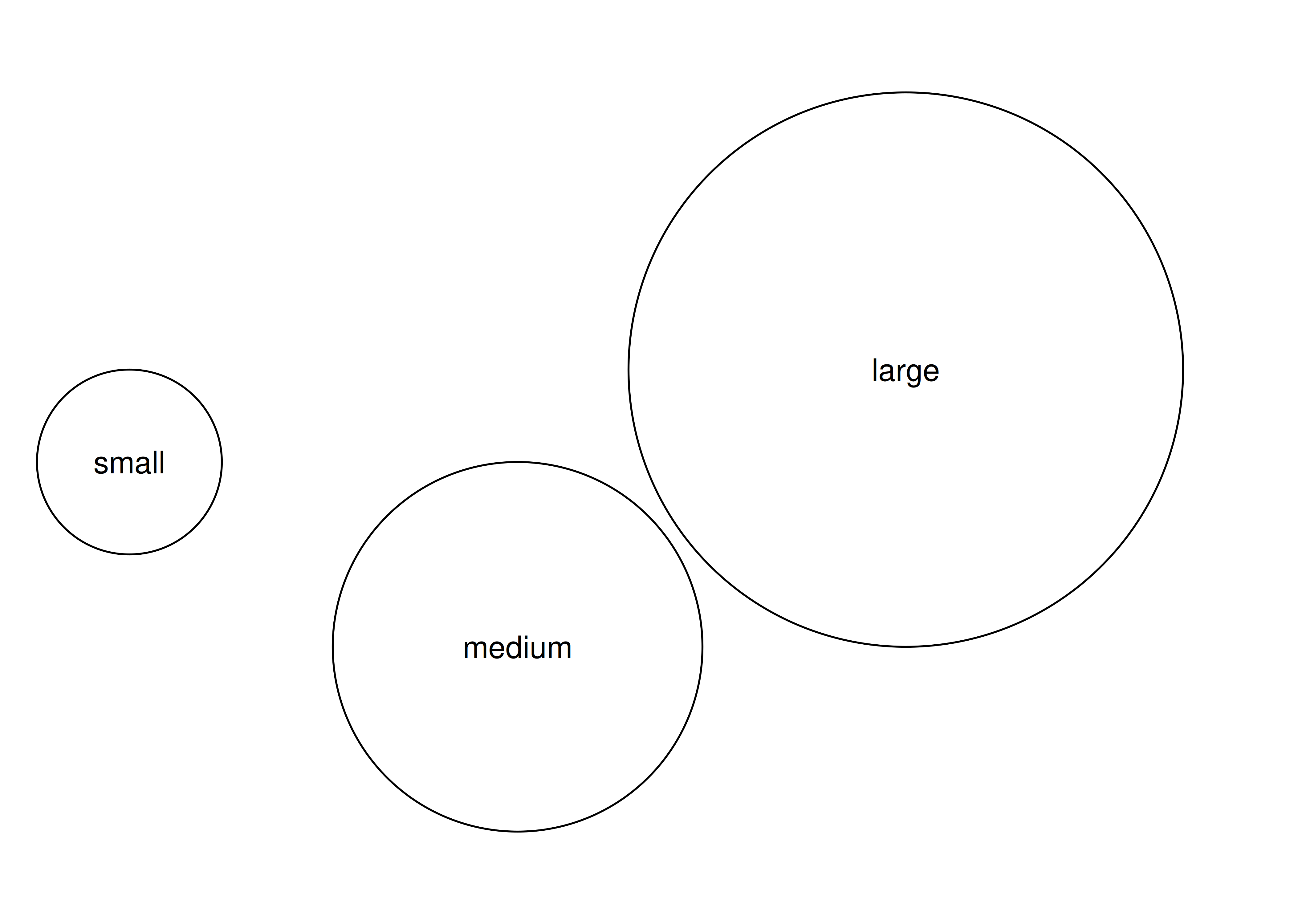It appears to work and we can now design our new (and final) geom. ### 22.5.3 The last GeomSpring GeomSpring <- ggproto("GeomSpring", Geom, setup_params = function(data, params) { if (is.null(params$n)) {
params$n <- 50 } else if (params$n <= 0) {
rlang::abort("Springs must be defined with n greater than 0")
}
params
},
draw_panel = function(data, panel_params, coord, n = 50, lineend = "butt",
na.rm = FALSE) {
data <- remove_missing(data, na.rm = na.rm,
c("x", "y", "xend", "yend", "linetype", "linewidth"),
name = "geom_spring")
if (is.null(data) || nrow(data) == 0) return(zeroGrob())
if (!coord$is_linear()) { rlang::warn("spring geom only works correctly on linear coordinate systems") } coord <- coord$transform(data, panel_params)
return(springGrob(coord$x, coord$y, coord$xend, coord$yend,
default.units = "native", diameter = unit(coord$diameter, "cm"), tension = coord$tension, n = n,
gp = gpar(
col = alpha(coord$colour, coord$alpha),
lwd = coord$linewidth * .pt, lty = coord$linetype,
lineend = lineend
)
))
},
required_aes = c("x", "y", "xend", "yend"),
default_aes = aes(
colour = "black",
linewidth = 0.5,
linetype = 1L,
alpha = NA,
diameter = 0.35,
tension = 0.75
)
)
geom_spring <- function(mapping = NULL, data = NULL, stat = "identity",
position = "identity", ..., n = 50, lineend = "butt",
na.rm = FALSE, show.legend = NA, inherit.aes = TRUE) {
layer(
data = data,
mapping = mapping,
stat = stat,
geom = GeomSpring,
position = position,
show.legend = show.legend,
inherit.aes = inherit.aes,
params = list(
n = n,
lineend = lineend,
na.rm = na.rm,
...
)
)
}

The main differences from our last GeomSpring implementation is that we no longer care about a group column because each spring is defined in one line, and then of course the draw_panel() method. Since we are no longer passing on the call to another geoms’ draw_panel() method we have additional obligations in that call. If the coordinate system is non-linear (e.g. coord_polar()) we emit a warning because our spring will not be adapted to that coordinate system. We then use the coordinate system to rescale our positional aesthetics with the transform() method. This will remap all positional aesthetics to lie between 0 and 1, with 0 being the lowest value visible in our viewport (scale expansions included) and 1 being the highest. With this remapping the coordinates are ready to be passed into a grob as "npc" units. By definition we understand the provided diameter as being given in centimeters. With all the values properly converted we call the springGrob() constructor and return the resulting grob. One thing we haven’t touched upon is the gpar() call inside the springGrob() construction. grid operates with a short list of very well-defined visual characteristics for grobs that are given by the gp argument in the constructor. This takes a gpar object that holds information such as colour of the stroke and fill, linetype, font, size, etc. Not all grobs care about all entries in gpar() and since we are constructing a line we only care about the gpar entries that the pathGrob understands, namely: col (stroke colour), lwd (line width), lty (line type), lineend (the terminator shape of the line).

ggplot(some_data) +
geom_spring(aes(
x = x,
y = y,
xend = xend,
yend = yend,
diameter = diameter,
tension = tension
))As can be seen in the example above we now have springs that do not shear with the aspect ratio of the plot and thus look to conform at every angle and aspect ratio. Further, resizing the plot will result in recalculations of the correct path so that it will continue to look as it should.

### 22.5.4 Post-Mortem

We have finally arrived at the spring geom we set out to make. The diameter of the spring behaves in the same way as a line width in that it remains fixed when resizing and/or changing the aspect ratio of the plot. There are still improvements we could (and perhaps, should) do to our geom. Most notably our create_spring() function remains un-vectorised and needs to be called for each spring separately. Correctly vectorizing this function will allow for considerable speed-up when rendering many springs (if that was ever a need). We will leave this as an exercise for the reader.

While the geom is now done, we still have a little work to do. We need to create a diameter scale and provide legend keys that can correctly communicate diameter and tension. This will be the topic of the final section.

## 22.6 Part 5: Scales

Now that we have our final geom, there’s still a bit of work to do before we are done. This is because we have defined a couple of new aesthetics in the process and we would like users to be able to scale them. There’s nothing wrong with defining new aesthetics without providing a scale — that simply means that the mapped values are passed through unchanged — but if we want users to have some control as well as the possibility of a legend we will need to provide scales for the aesthetics. This will be the goal of this final section.

### 22.6.1 Scaling

Thankfully, compared to grid, creating new scales is not a huge undertaking. It basically surmounts to creating a function with the correct name that outputs a Scale object. In the code below you can see how this is done for the tension aesthetic:

scale_tension_continuous <- function(..., range = c(0.1, 1)) {
continuous_scale(
aesthetics = "tension",
scale_name = "tension_c",
palette = scales::rescale_pal(range),
...
)
}

Most scale functions are simply wrappers around calls to one of the scale constructors (continuous_scale(), discrete_scale(), and binned_scale()). Most importantly it names the aesthetic(s) this scale relates to and provides a palette function which transforms the input domain to the output range. All the remaining well-known arguments from scale functions such as name, breaks, limits, etc. are carried through with the .... For cases such as these where only a single scale is relevant for an aesthetic you’ll often create a short-named version as well. We’ll also add a discrete scale to catch if this aesthetic is erroneously being used with discrete data:

scale_tension <- scale_tension_continuous

scale_tension_discrete <- function(...) {
rlang::abort("Tension cannot be used with discrete data")
}

The reason why we need scale_tension_continuous() when we also have scale_tension() is that the default scale for aesthetics is looked up by searching for a function called scale_<aesthetic-name>_<data-type>.

While we are at it we’ll create a scale for the diameter as well:

scale_diameter_continuous <- function(..., range = c(0.25, 0.7), unit = "cm") {
range <- grid::convertWidth(unit(range, unit), "cm", valueOnly = TRUE)
continuous_scale(
aesthetics = "diameter",
scale_name = "diameter_c",
palette = scales::rescale_pal(range),
...
)
}
scale_diameter <- scale_diameter_continuous
scale_tension_discrete <- function(...) {
rlang::abort("Diameter cannot be used with discrete data")
}

The only change we made from the tension scales is that we allow the user to define which unit the diameter range should be measured in. Since the geom expects centimeters we will convert the range to that before passing it into the scale constructor. In that way the user is free to use whatever absolute unit feels natural to them.

With our scales defined let us have a look:

ggplot(some_data) +
geom_spring(aes(x = x, y = y, xend = xend, yend = yend, tension = tension,
diameter = diameter)) +
scale_tension(range = c(0.1, 5))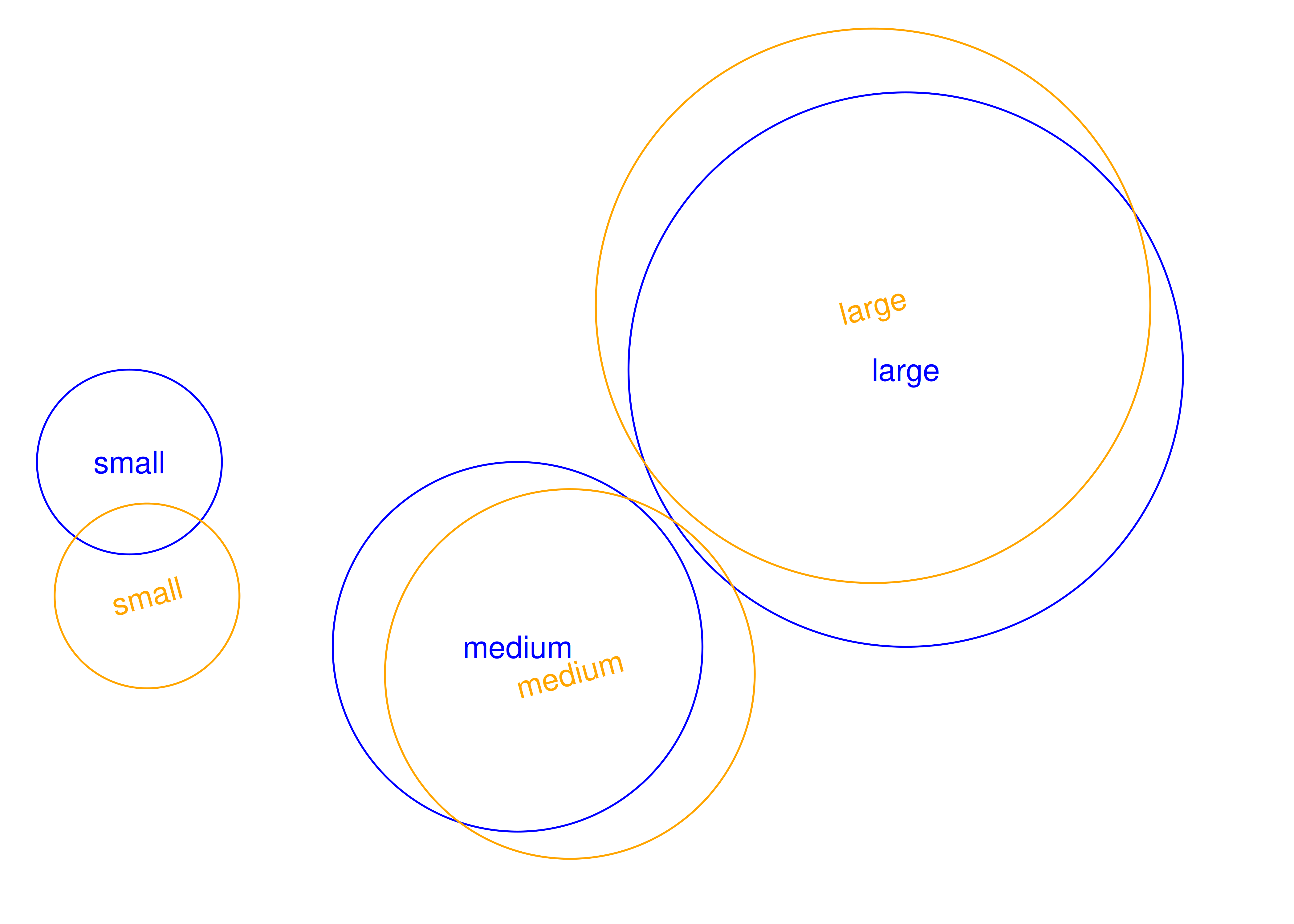The code above shows us that both the default scale (we didn’t add an explicit scale for diameter) and the custom scales (scale_tension()) work. It also tells us that our job is not done, because the legend is pretty uninformative. That is because our geom uses the default legend key constructor which is draw_key_point(). This key constructor doesn’t know what to do about our new aesthetics and ignores it completely.

### 22.6.2 draw_key_spring

The key constructors are pretty simple constructors that take a data.frame of aesthetic values and uses that to draw a given representation. If we look at the point key constructor we see that it simply constructs a pointsGrob:

draw_key_point
#> function (data, params, size)
#> {
#>     if (is.null(data$shape)) { #> data$shape <- 19
#>     }
#>     else if (is.character(data$shape)) { #> data$shape <- translate_shape_string(data$shape) #> } #> stroke_size <- data$stroke %||% 0.5
#>     stroke_size[is.na(stroke_size)] <- 0
#>     pointsGrob(0.5, 0.5, pch = data$shape, gp = gpar(col = alpha(data$colour %||%
#>         "black", data$alpha), fill = alpha(data$fill %||% "black",
#>         data$alpha), fontsize = (data$size %||% 1.5) * .pt +
#>         stroke_size * .stroke/2, lwd = stroke_size * .stroke/2))
#> }
#> <bytecode: 0x55eff7eb62d8>
#> <environment: namespace:ggplot2>

data is a data.frame with a single row giving the aesthetic values to use for the key, params are the geom params for the layer, and size is the size of the key area in centimeters.

To create one that matches well with our new geom we should simply try to create a key that uses our springGrob instead:

draw_key_spring <- function(data, params, size) {
springGrob(
x0 = 0, y0 = 0, x1 = 1, y1 = 1,
diameter = unit(data$diameter, "cm"), tension = data$tension,
gp = gpar(
col = alpha(data$colour %||% "black", data$alpha),
lwd = (data$size %||% 0.5) * .pt, lty = data$linetype %||% 1
),
vp = viewport(clip = "on")
)
} 

We add a little flourish here that is not necessary for the point key constructor, which is that we define a clipping viewport for our grob. This means that the spring will not spill-out into the neighboring keys.

Along with that we will also have to modify our Geom to use this key constructor instead (We know we said the last version was final). We don’t have to define our Geom from scratch again, though but simply change the draw_key() method of our existing Geom:

GeomSpring\$draw_key <- draw_key_spring

With that final change our legend is beginning to make sense:

ggplot(some_data) +
geom_spring(aes(x = x, y = y, xend = xend, yend = yend, tension = tension,
diameter = diameter)) +
scale_tension(range = c(0.1, 5))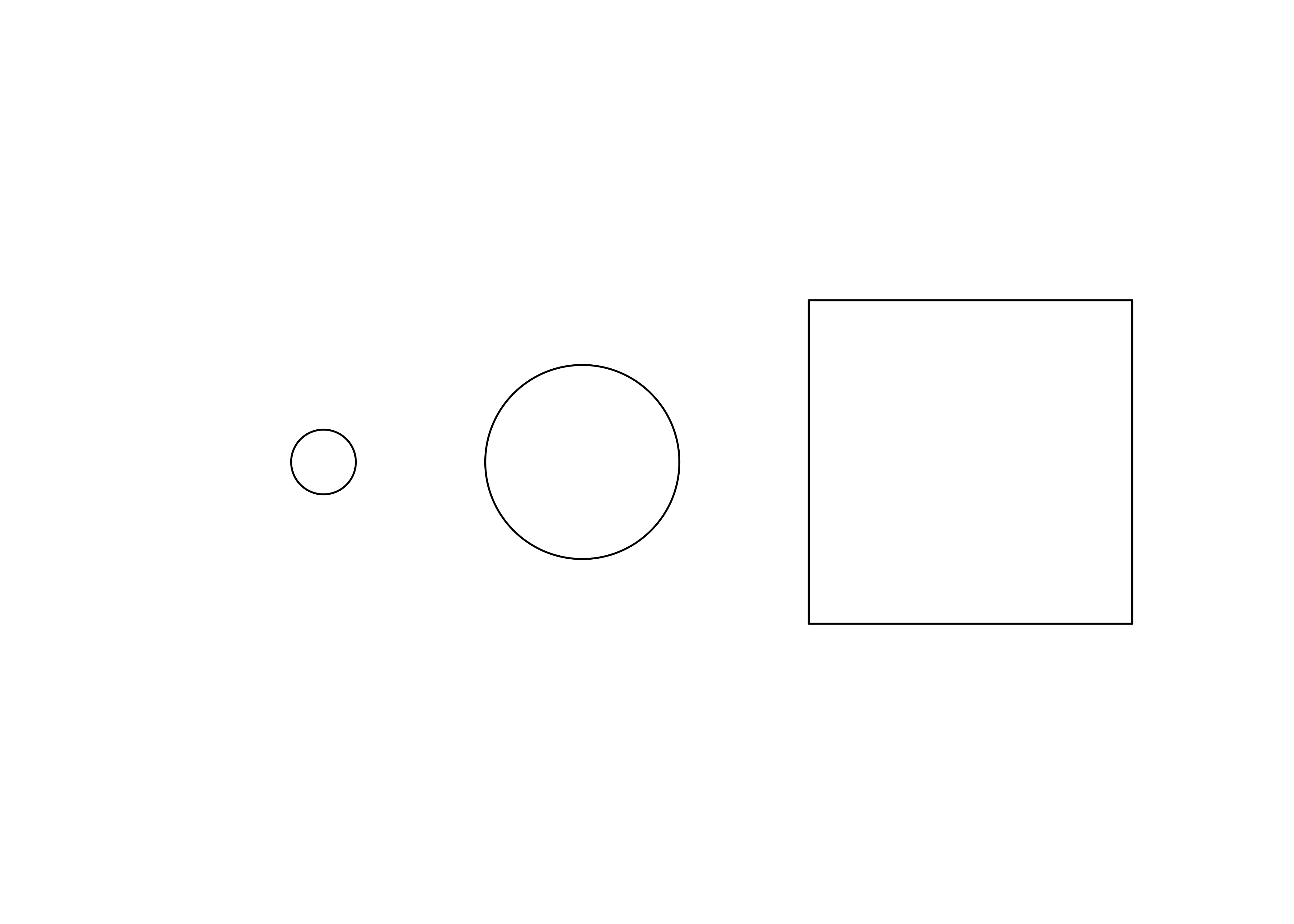The default key size is a bit cramped for our key, but that has to be modified by the user (ggplot2 doesn’t know about the diameter aesthetic and cannot scale the key size to that in the same way as it does with the size aesthetic).

ggplot(some_data) +
geom_spring(aes(x = x, y = y, xend = xend, yend = yend, tension = tension,
diameter = diameter)) +
scale_tension(range = c(0.1, 5)) +
theme(legend.key.size = unit(1, "cm"))The new legend key will be used for all scaled aesthetics, not just our new diameter and tension meaning that the key will always match the style of the layer:

ggplot(some_data) +
geom_spring(aes(x = x, y = y, xend = xend, yend = yend, colour = class)) +
theme(legend.key.size = unit(1, "cm"))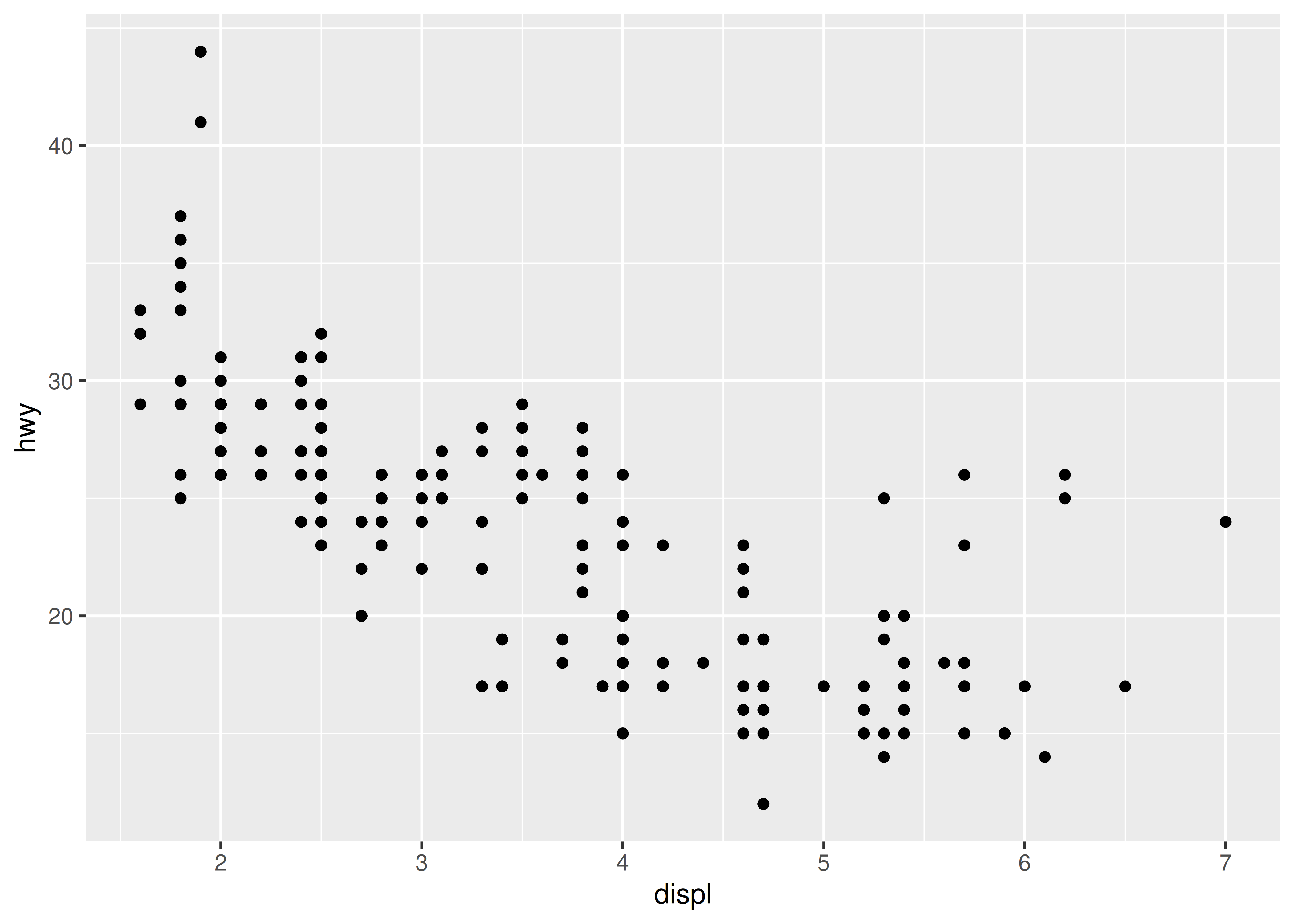### 22.6.3 Post-Mortem

This concludes our, admittedly a bit far-fetched, case study on how to create a spring geom. Hopefully it has become clear that there are many different ways to achieve the same geom extension and where you end up is largely guided by your needs and how much energy you want to put into it. While extending layers (and scales) are only a single (but important) part of the ggplot2 extension system, we will not discuss how to create other types of extensions such as coord and facet extensions. The curious reader is invited to study the source code of both ggplot2’s own Facet and Coord classes as well as the extensions available in e.g. the ggforce package.